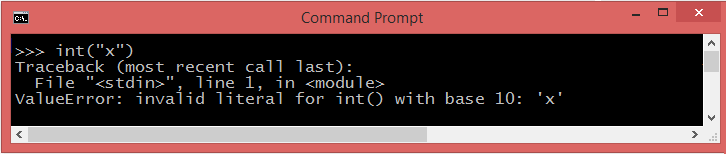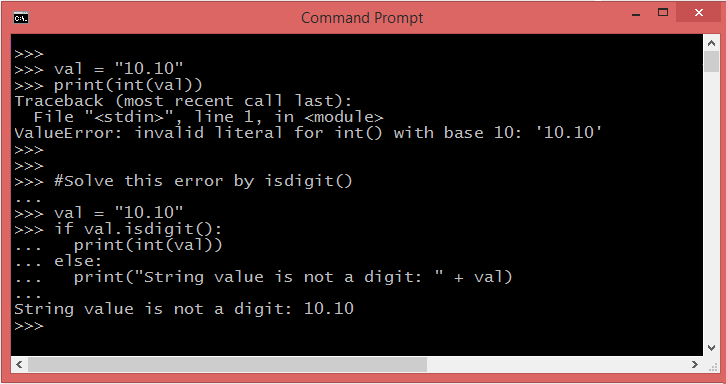# invalid literal for int() with base 10

The error message invalid literal for int() with base 10 would seem to indicate that you are passing a string that's not an integer to the int() function . In other words it's either empty, or has a character in it other than a digit.## int() method

The int() method is the python's inbuilt function which converts the given number or string into an integer . The default base is 10. This method return an integer object constructed from a number or string, or return 0 if no arguments are given.But you get a ValueError: invalid literal for int() with base 10 , if you pass a string representation of a float into int , or a string representation of anything but an integer (including empty string).

## How to fix it?

### Python isdigit()

You can solve this error by using Python isdigit() method to check whether the value is number or not. The returns True if all the characters are digits, otherwise False .
val = "10.10" if val.isdigit(): print(int(val))### Using try-except

The other way to overcome this issue is to wrap your code inside a Python try...except block to handle this error.
str ='noninteger' try: int(str) except: print('Can not convert', str ,"to int")

### Floating-Point Numbers

If you are trying to convert a float string (eg. "10.10") to an integer, simply calling float first then converting that to an int will work:
output = int(float(input))The above code converts the string ("10.10") to a floating point value, which is then converted to an integer via truncation—that is, by discarding the fractional part . Applying these functions to "10.10" will produce a result of 10. If, on the other hand, you wanted the floating point value , just use only float().

## Python2.x and Python3.x

Sometimes the difference between Python2.x and Python3.x that leads to this ValueError: invalid literal for int() with base 10 . With Python2.x , int(str(3/2)) gives you "1". With Python3.x , the same gives you ("1.5"): ValueError: invalid literal for int() with base 10: "1.5".

Python is pretty good at abstracting this away from you, most other language also have double precision floating point numbers, for instance, but you don't need to worry about that. Since 3.0, Python will also automatically convert integers to floats if you divide them, so it's actually very easy to work with.

Computers store numbers in a variety of different ways. Python has two main ones. Integers, which store whole numbers ie integers, and floating point numbers, which store real numbers . "base 10" means that you count from 0 to 9. You need to use the right one based on what you require.